# Contents

cranpkgs=c("ggplot2","devtools","adaptiveGPCA","treelapse", "ade4","structSSI", "PMA","vegan", "ggrepel","gridExtra")
BioCpkgs=c("dada2", "curatedMetagenomicData", "phyloseq", "DECIPHER","genefilter")
ghpkgs= c("treeDA", "treelapse","metavizr")
instp <-  cranpkgs %in% installed.packages()
if(any(!instp)) {
install.packages(cranpkgs[!instp],repos="https://cloud.r-project.org")
}
instp <- BioCpkgs %in% installed.packages()
if(any(!instp)) {
source("http://bioconductor.org/biocLite.R")
biocLite(BioCpkgs [!instp], ask = F)
}

if(!("treeDA"  %in% installed.packages()))
BiocInstaller::biocLite("jfukuyama/treeDA", dependencies = TRUE)
if(!("treelapse"  %in% installed.packages()))
BiocInstaller::biocLite("krisrs1128/treelapse", dependencies = TRUE)

suppressPackageStartupMessages(sapply(c(cranpkgs, BioCpkgs, ghpkgs), require, character.only = TRUE))
##                ggplot2               devtools           adaptiveGPCA              treelapse
##                   TRUE                   TRUE                   TRUE                   TRUE
##                   ade4              structSSI                    PMA                  vegan
##                   TRUE                   TRUE                   TRUE                   TRUE
##                ggrepel              gridExtra                  dada2 curatedMetagenomicData
##                   TRUE                   TRUE                   TRUE                   TRUE
##               phyloseq               DECIPHER             genefilter                 treeDA
##                   TRUE                   TRUE                   TRUE                   TRUE
##              treelapse               metavizr
##                   TRUE                   TRUE

# dada2 theory

The microbiome is formed of the ecological communities of microorganisms that dominate the living world. Bacteria can now be identified through the use of next generation sequencing applied at several levels. Shotgun sequencing of all bacteria in a sample delivers knowledge of all the genes present. Here we will only be interested in the identification and quantification of individual taxa (or species) through a ‘fingerprint gene’ called 16s rRNA which is present in all bacteria. This gene presents several variable regions which can be used to identify the different taxa.

Previous standard workflows depended on clustering all 16s rRNA sequences (generated by next generation amplicon sequencing) that occur within a 97% radius of similarity and then assigning these to ‘OTUs’ from reference trees (Caporaso et al. 2010; Schloss et al. 2009). These approaches do not incorporate all the data, in particular sequence quality information and statistical information available on the reads were not incorporated into the assignments.

In contrast, the de novo read counts used here will be constructed through the incorporation of both the quality scores and sequence frequencies in a probabilistic noise model for nucleotide transitions. For more details on the algorithmic implementation of this step see (Benjamin J Callahan et al. 2016).

After filtering the sequences and removing the chimeræ, the data are compared to a standard database of bacteria and labeled. In this workflow, we have used the labeled sequences to build a de novo phylogenetic with the .

The key step in the sequence analysis is the manner in which reads are denoised and assembled into groups we have chosen to call ASVs (Amplicon Sequence Variants)(Callahan, McMurdie, and Holmes 2017) instead of the traditional OTUs(Operational Taxonomic Units).

A published (but essentially similar) version of this workflow, including reviewer reports and comments is available (Ben J Callahan et al. 2016), see F1000Research From Raw reads.

This is a workflow for denoising, filtering, performing data transformations, visualization, supervised learning analyses, community network tests, hierarchical testing and linear models. We provide all the code and give several examples of different types of analyses and use-cases. There are often many different objectives in experiments involving microbiome data and we will only give a flavor for what could be possible once the data has been imported into R.

In addition, the code can be easily adapted to accommodate batch effects, covariates and multiple experimental factors.

This workflow is based on software packages from the open-source Bioconductor project (Huber et al. 2015). We provide all steps necessary from the denoising and identification of the reads input as raw sequences as fastq files to the comparative testing and multivariate analyses of the samples and analyses of the abundances according to multiple available covariates.

# dada2 tutorial

See tutorial here

We will select portions of Complete Bioconductor Worflow output to cover in this tutorial

# Using phyloseq

phyloseq(McMurdie and Holmes 2013) is an R package to import, store, analyze, and graphically display complex phylogenetic sequencing data that has already been clustered into Operational Taxonomic Units (OTUs) or more appropriately denoised, and it is most useful when there is also associated sample data, phylogeny, and/or taxonomic assignment of each taxa. leverages and builds upon many of the tools available in R for ecology and phylogenetic analysis (ape, vegan, ade4), while also using advanced/flexible graphic systems (ggplot2) to easily produce publication-quality graphics of complex phylogenetic data. The phyloseq package uses a specialized system of S4 data classes to store all related phylogenetic sequencing data as a single, self-consistent, self-describing experiment-level object, making it easier to share data and reproduce analyses. In general, phyloseq seeks to facilitate the use of R for efficient interactive and reproducible analysis of amplicon count data jointly with important sample covariates.

This tutorial shows a useful example workflow, but many more analyses are available to you in phyloseq, and R in general, than can fit in a single workflow. The phyloseq home page is a good place to begin browsing additional phyloseq documentation, as are the three vignettes included within the package, and linked directly at the phyloseq release page on Bioconductor.

## Loading the data

Many use cases result in the need to import and combine different data into a phyloseq class object, this can be done using th import_biom function to read recent QIIME format files, older files can still be imported with import_qiime. More complete details can be found on the phyloseq FAQ page.

In the previous section the results of dada2 sequence processing were organized into a phyloseq object. We have actually run dada2 on a larger set of samples from the same data source. This object was also saved in R-native serialized RDS format. We will re-load this here for completeness as the initial object ps. If you have not downloaded the whole repository you can access the ps file though github:

ps_connect <-url("https://raw.githubusercontent.com/spholmes/F1000_workflow/master/data/ps.rds")
ps = readRDS(ps_connect)
ps
## phyloseq-class experiment-level object
## otu_table()   OTU Table:         [ 389 taxa and 360 samples ]
## sample_data() Sample Data:       [ 360 samples by 14 sample variables ]
## tax_table()   Taxonomy Table:    [ 389 taxa by 6 taxonomic ranks ]
## phy_tree()    Phylogenetic Tree: [ 389 tips and 387 internal nodes ]

## Shiny-phyloseq

It can be beneficial to start the data exploration process interactively, this often saves time in detecting outliers and specific features of the data. Shiny-phyloseq (McMurdie and Holmes 2015) is an interactive web application that provides a graphical user interface to the phyloseq package. The object just loaded into the R session in this workflow is suitable for graphical exploration with Shiny-phyloseq.

## Filtering

phyloseq provides useful tools for filtering, subsetting, and agglomerating taxa – a task that is often appropriate or even necessary for effective analysis of microbiome count data. In this subsection, we graphically explore the prevalence of taxa in the example dataset, and demonstrate how this can be used as a filtering criteria. One of the reasons to filter in this way is to avoid spending much time analyzing taxa that were seen only rarely among samples. This also turns out to be a useful filter of noise (taxa that are actually just artifacts of the data collection process), a step that should probably be considered essential for datasets constructed via heuristic OTU-clustering methods, which are notoriously prone to generating spurious taxa.

### Taxonomic Filtering

In many biological settings, the set of all organisms from all samples are well-represented in the available taxonomic reference database. When (and only when) this is the case, it is reasonable or even advisable to filter taxonomic features for which a high-rank taxonomy could not be assigned. Such ambiguous features in this setting are almost always sequence artifacts that don’t exist in nature. It should be obvious that such a filter is not appropriate for samples from poorly characterized or novel specimens, at least until the possibility of taxonomic novelty can be satisfactorily rejected. Phylum is a useful taxonomic rank to consider using for this purpose, but others may work effectively for your data.

To begin, create a table of read counts for each Phylum present in the dataset.

# Show available ranks in the dataset
rank_names(ps)
##  "Kingdom" "Phylum"  "Class"   "Order"   "Family"  "Genus"
# Create table, number of features for each phyla
table(tax_table(ps)[, "Phylum"], exclude = NULL)
##
##              Actinobacteria               Bacteroidetes Candidatus_Saccharibacteria
##                          13                          23                           1
##   Cyanobacteria/Chloroplast         Deinococcus-Thermus                  Firmicutes
##                           4                           1                         327
##                Fusobacteria              Proteobacteria                 Tenericutes
##                           1                          11                           1
##             Verrucomicrobia                        <NA>
##                           1                           6

This shows a few phyla for which only one feature was observed. Those may be worth filtering, and we’ll check that next. First, notice that in this case, six features were annotated with a Phylum of NA. These features are probably artifacts in a dataset like this, and should be removed.

The following ensures that features with ambiguous phylum annotation are also removed. Note the flexibility in defining strings that should be considered ambiguous annotation.

ps <- subset_taxa(ps, !is.na(Phylum) & !Phylum %in% c("", "uncharacterized"))

A useful next step is to explore feature prevalence in the dataset, which we will define here as the number of samples in which a taxon appears at least once.

# Compute prevalence of each feature, store as data.frame
prevdf = apply(X = otu_table(ps),
MARGIN = ifelse(taxa_are_rows(ps), yes = 1, no = 2),
FUN = function(x){sum(x > 0)})
# Add taxonomy and total read counts to this data.frame
prevdf = data.frame(Prevalence = prevdf,
TotalAbundance = taxa_sums(ps),
tax_table(ps))

Are there phyla that are comprised of mostly low-prevalence features? Compute the total and average prevalences of the features in each phylum.

plyr::ddply(prevdf, "Phylum", function(df1){cbind(mean(df1$Prevalence),sum(df1$Prevalence))})
##                         Phylum         1     2
## 1               Actinobacteria 120.15385  1562
## 2                Bacteroidetes 265.52174  6107
## 3  Candidatus_Saccharibacteria 280.00000   280
## 4    Cyanobacteria/Chloroplast  64.25000   257
## 5          Deinococcus-Thermus  52.00000    52
## 6                   Firmicutes 179.24771 58614
## 7                 Fusobacteria   2.00000     2
## 8               Proteobacteria  59.09091   650
## 9                  Tenericutes 234.00000   234
## 10             Verrucomicrobia 104.00000   104

Deinococcus-Thermus appeared in just over one percent of samples, and Fusobacteria appeared in just 2 samples total. In some cases it might be worthwhile to explore these two phyla in more detail despite this (though probably not Fusobacteria’s two samples). For the purposes of this example, though, they will be filtered from the dataset.

# Define phyla to filter
filterPhyla = c("Fusobacteria", "Deinococcus-Thermus")
# Filter entries with unidentified Phylum.
ps1 = subset_taxa(ps, !Phylum %in% filterPhyla)
ps1
## phyloseq-class experiment-level object
## otu_table()   OTU Table:         [ 381 taxa and 360 samples ]
## sample_data() Sample Data:       [ 360 samples by 14 sample variables ]
## tax_table()   Taxonomy Table:    [ 381 taxa by 6 taxonomic ranks ]
## phy_tree()    Phylogenetic Tree: [ 381 tips and 379 internal nodes ]

### Prevalence Filtering

The previous filtering steps are considered supervised, because they relied on prior information that is external to this experiment (a taxonomic reference database). This next filtering step is completely unsupervised, relying only on the data in this experiment, and a parameter that we will choose after exploring the data. Thus, this filtering step can be applied even in settings where taxonomic annotation is unavailable or unreliable.

First, explore the relationship of prevalence and total read count for each feature. Sometimes this reveals outliers that should probably be removed, and also provides insight into the ranges of either feature that might be useful. This aspect depends quite a lot on the experimental design and goals of the downstream inference, so keep these in mind. It may even be the case that different types of downstream inference require different choices here. There is no reason to expect ahead of time that a single filtering workflow is appropriate for all analysis.

# Subset to the remaining phyla
prevdf1 = subset(prevdf, Phylum %in% get_taxa_unique(ps1, "Phylum"))
ggplot(prevdf1, aes(TotalAbundance, Prevalence / nsamples(ps),color=Phylum)) +
# Include a guess for parameter
geom_hline(yintercept = 0.05, alpha = 0.5, linetype = 2) +  geom_point(size = 2, alpha = 0.7) +
scale_x_log10() +  xlab("Total Abundance") + ylab("Prevalence [Frac. Samples]") +
facet_wrap(~Phylum) + theme(legend.position="none")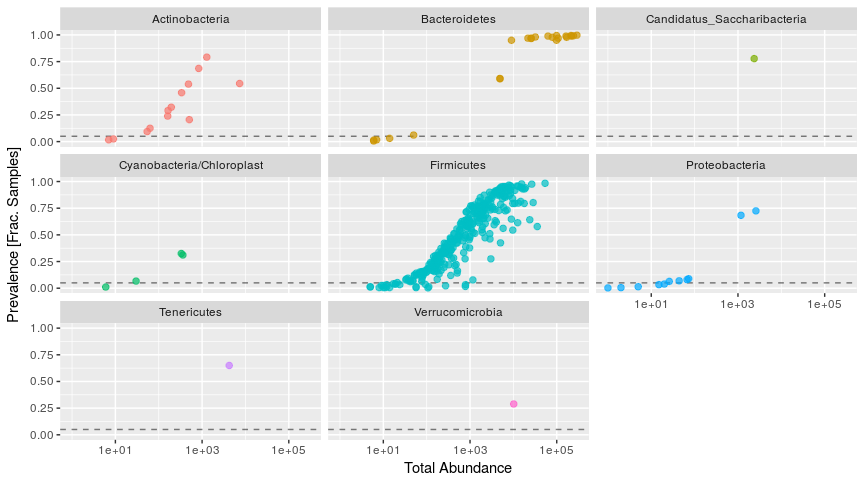Each point in Figure @ref(fig:plotprevalence) is a different taxa. Exploration of the data in this way is often useful for selecting filtering parameters, like the minimum prevalence criteria we will used to filter the data above.

Sometimes a natural separation in the dataset reveals itself, or at least, a conservative choice that is in a stable region for which small changes to the choice would have minor or no effect on the biological interpreation (stability). Here no natural separation is immediately evident, but it looks like we might reasonably define a prevalence threshold in a range of zero to ten percent or so. Take care that this choice does not introduce bias into a downstream analysis of association of differential abundance.

The following uses five percent of all samples as the prevalence threshold.

# Define prevalence threshold as 5% of total samples
prevalenceThreshold = 0.05 * nsamples(ps)
prevalenceThreshold
##  18
# Execute prevalence filter, using prune_taxa() function
keepTaxa = rownames(prevdf1)[(prevdf1$Prevalence >= prevalenceThreshold)] ps2 = prune_taxa(keepTaxa, ps) ## Agglomerate taxa When there is known to be a lot of species or sub-species functional redundancy in a microbial community, it might be useful to agglomerate the data features corresponding to closely related taxa. Ideally we would know the functional redundancies perfectly ahead of time, in which case we would agglomerate taxa using those defined relationships and the function in phyloseq. That kind of exquisite functional data is usually not available, and different pairs of microbes will have different sets of overlapping functions, complicating the matter of defining appropriate grouping criteria. While not necessarily the most useful or functionally-accurate criteria for grouping microbial features (sometimes far from accurate), taxonomic agglomeration has the advantage of being much easier to define ahead of time. This is because taxonomies are usually defined with a comparatively simple tree-like graph structure that has a fixed number of internal nodes, called “ranks”. This structure is simple enough for the phyloseq package to represent taxonomies as table of taxonomy labels. Taxonomic agglomeration groups all the “leaves” in the hierarchy that descend from the user-prescribed agglomerating rank, this is sometimes called ‘glomming’. The following example code shows how one would combine all features that descend from the same genus. # How many genera would be present after filtering? length(get_taxa_unique(ps2, taxonomic.rank = "Genus")) ##  49 ps3 = tax_glom(ps2, "Genus", NArm = TRUE) If taxonomy is not available or not reliable, tree-based agglomeration is a “taxonomy-free” alternative to combine data features corresponding to closely-related taxa. In this case, rather than taxonomic rank, the user specifies a tree height corresponding to the phylogenetic distance between features that should define their grouping. This is very similar to “OTU Clustering”, except that in many OTU Clustering algorithms the sequence distance being used does not have the same (or any) evolutionary definition. h1 = 0.4 ps4 = tip_glom(ps2, h = h1) Here phyloseq’s plot_tree() function compare the original unfiltered data, the tree after taxonoic agglomeration, and the tree after phylogenetic agglomeration. These are stored as separate plot objects, then rendered together in one combined graphic using gridExtra::grid.arrange. multiPlotTitleTextSize = 15 p2tree = plot_tree(ps2, method = "treeonly", ladderize = "left", title = "Before Agglomeration") + theme(plot.title = element_text(size = multiPlotTitleTextSize)) p3tree = plot_tree(ps3, method = "treeonly", ladderize = "left", title = "By Genus") + theme(plot.title = element_text(size = multiPlotTitleTextSize)) p4tree = plot_tree(ps4, method = "treeonly", ladderize = "left", title = "By Height") + theme(plot.title = element_text(size = multiPlotTitleTextSize)) # group plots together grid.arrange(nrow = 1, p2tree, p3tree, p4tree)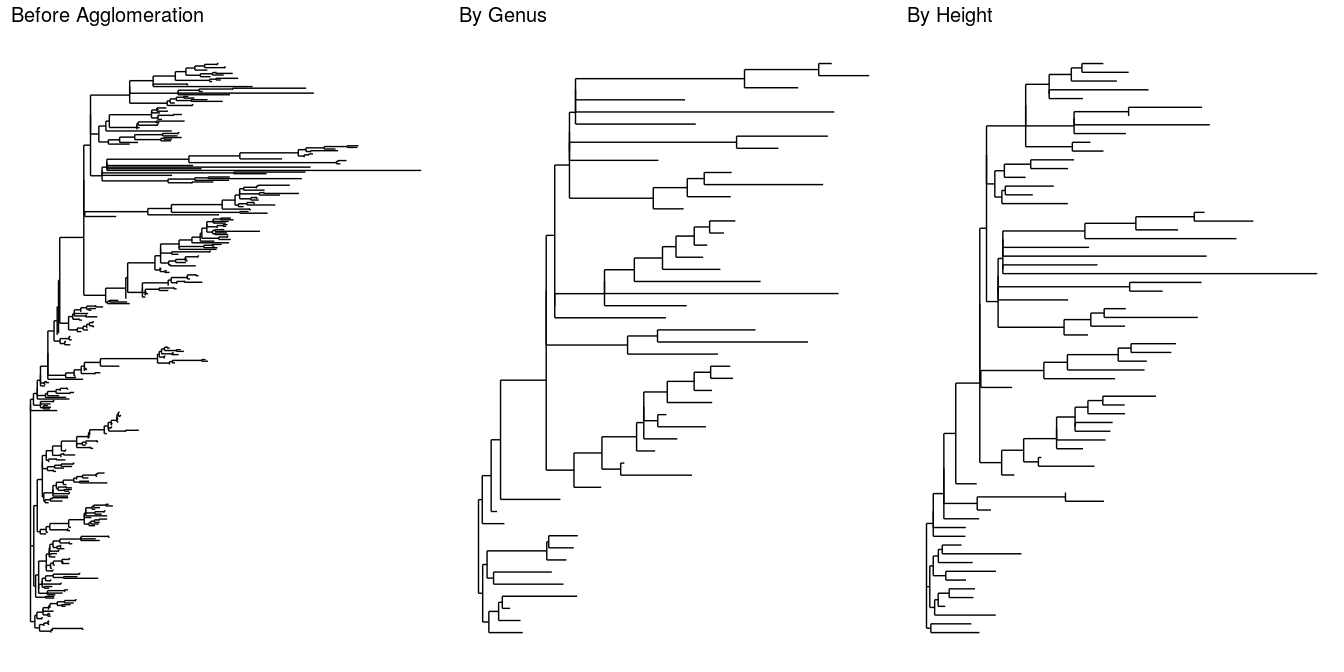Figure @ref(fig:plotglomtree) shows the original tree on the left, taxonomic agglomeration at Genus rank in the middle and phylogenetic agglomeration at a fixed distance of 0.4 on the right. ## Abundance value transformation It is usually necessary to transform microbiome count data to account for differences in library size, variance, scale, etc. The phyloseq package provides a flexible interface for defining new functions to accomplish these transformations of the abundance values via the function transform_sample_counts(). The first argument to this function is the phyloseq object you want to transform, and the second argument is an R function that defines the transformation. The R function is applied sample-wise, expecting that the first unnamed argument is a vector of taxa counts in the same order as the phyloseq object. Additional arguments are passed on to the function specified in the second argument, providing an explicit means to include pre-computed values, previously defined parameters/thresholds, or any other object that might be appropriate for computing the transformed values of interest. This example begins by defining a custom plot function, plot_abundance(), that uses phyloseq’s function to define a relative abundance graphic. We will use this to compare more easily differences in scale and distribution of the abundance values in our phyloseq object before and after transformation. plot_abundance = function(physeq,title = "", Facet = "Order", Color = "Phylum"){ # Arbitrary subset, based on Phylum, for plotting p1f = subset_taxa(physeq, Phylum %in% c("Firmicutes")) mphyseq = psmelt(p1f) mphyseq <- subset(mphyseq, Abundance > 0) ggplot(data = mphyseq, mapping = aes_string(x = "sex",y = "Abundance", color = Color, fill = Color)) + geom_violin(fill = NA) + geom_point(size = 1, alpha = 0.3, position = position_jitter(width = 0.3)) + facet_wrap(facets = Facet) + scale_y_log10()+ theme(legend.position="none") } The transformation in this case converts the counts from each sample into their frequencies, often referred to as proportions or relative abundances. This function is so simple that it is easiest to define it within the function call to transform_sample_counts(). # Transform to relative abundance. Save as new object. ps3ra = transform_sample_counts(ps3, function(x){x / sum(x)}) Now we plot the abundance values before and after transformation. plotBefore = plot_abundance(ps3,"") plotAfter = plot_abundance(ps3ra,"") # Combine each plot into one graphic. grid.arrange(nrow = 2, plotBefore, plotAfter)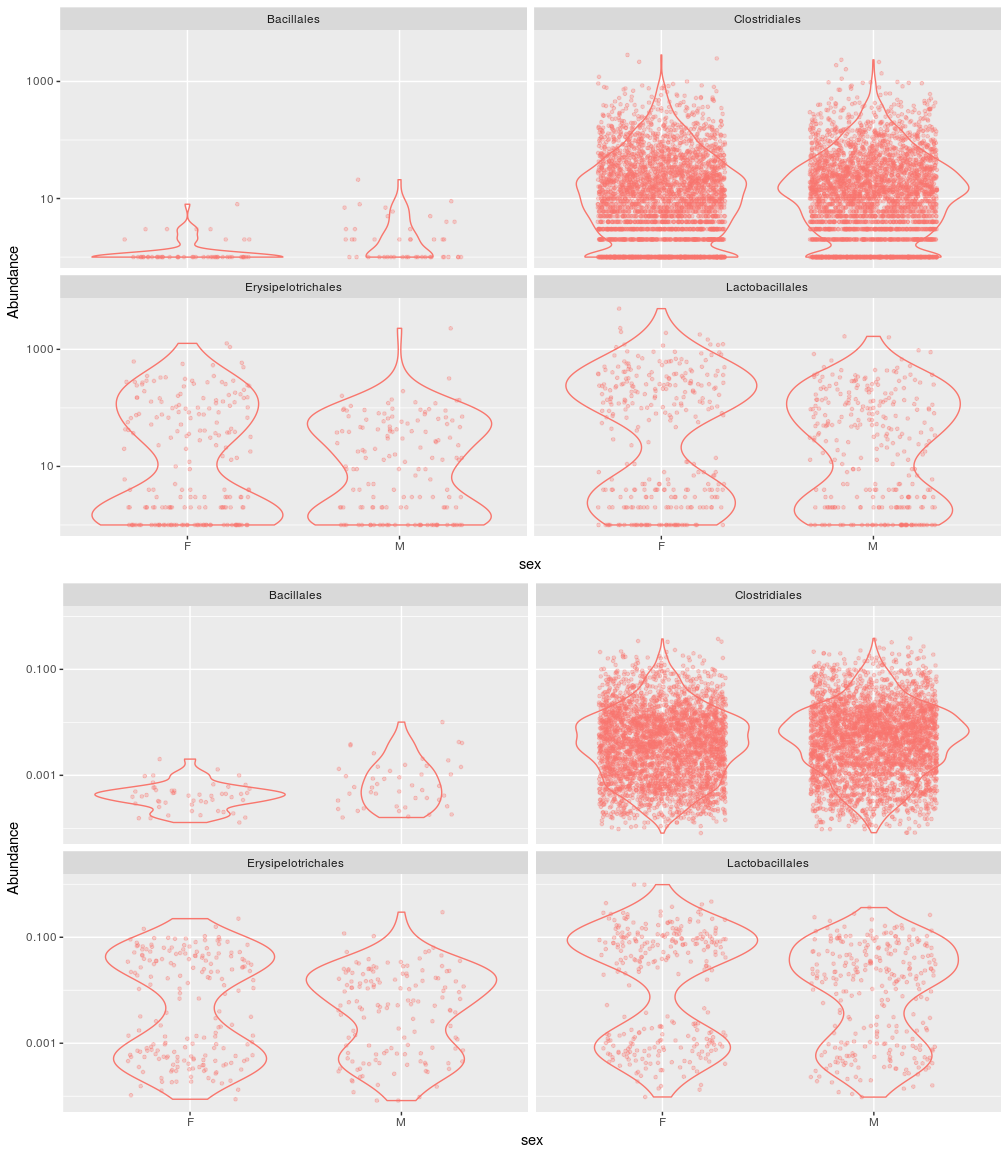Figure @ref(fig:abundancetransformation3) shows the comparison of original abundances (top panel) and relative abundances (lower). ## Subset by taxonomy Notice on the previous plot that Lactobacillales appears to be a taxonomic Order with bimodal abundance profile in the data. We can check for a taxonomic explanation of this pattern by plotting just that taxonomic subset of the data. For this, we subset with the function, and then specify a more precise taxonomic rank to the argument of the function that we defined above. psOrd = subset_taxa(ps3ra, Order == "Lactobacillales") plot_abundance(psOrd, Facet = "Genus", Color = NULL)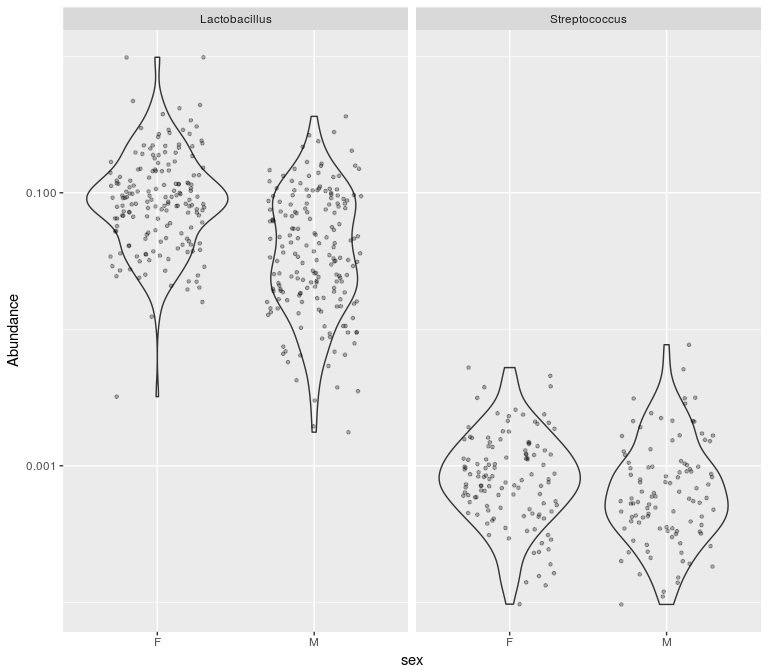Figure @ref(fig:subsettaxa) shows the relative abundances of Lactobacillales taxonomic Order, grouped by host sex and genera. Here it is clear that the apparent biomodal distribution of Lactobacillales on the previous plot was the result of a mixture of two different genera, with the typical Lactobacillus relative abundance much larger than Streptococcus. At this stage in the workflow, after converting raw reads to interpretable species abundances, and after filtering and transforming these abundances to focus attention on scientifically meaningful quantities, we are in a position to consider more careful statistical analysis. R is an ideal environment for performing these analyses, as it has an active community of package developers building simple interfaces to sophisticated techniques. As a variety of methods are available, there is no need to commit to any rigid analysis strategy a priori. Further, the ability to easily call packages without reimplementing methods frees researchers to iterate rapidly through alternative analysis ideas. The advantage of performing this full workflow in R is that this transition from bioinformatics to statistics is effortless. Let’s start by installing a few packages that are available for these complementary analyses: .cran_packages <- c( "shiny","miniUI", "caret", "pls", "e1071", "ggplot2", "randomForest", "dplyr", "ggrepel", "nlme", "devtools", "reshape2", "PMA", "structSSI", "ade4", "ggnetwork", "intergraph", "scales") .github_packages <- c("jfukuyama/phyloseqGraphTest") .bioc_packages <- c("genefilter", "impute") # Install CRAN packages (if not already installed) .inst <- .cran_packages %in% installed.packages() if (any(!.inst)){ install.packages(.cran_packages[!.inst],repos = "http://cran.rstudio.com/") } .inst <- .github_packages %in% installed.packages() if (any(!.inst)){ devtools::install_github(.github_packages[!.inst]) } .inst <- .bioc_packages %in% installed.packages() if(any(!.inst)){ source("http://bioconductor.org/biocLite.R") biocLite(.bioc_packages[!.inst]) } We back these claims by illustrating several analyses on the mouse data prepared above. We experiment with several flavors of exploratory ordination before shifting to more formal testing and modeling, explaining the settings in which the different points of view are most appropriate. Finally, we provide example analyses of multitable data, using a study in which both metabolomic and microbial abundance measurements were collected on the same samples, to demonstrate that the general workflow presented here can be adapted to the multitable setting. ### Preprocessing Before doing the multivariate projections, we will add a few columns to our sample data, which can then be used to annotate plots. From Figure @ref(fig:preprocessing-setup), we see that the ages of the mice come in a couple of groups, and so we make a categorical variable corresponding to young, middle-aged, and old mice. We also record the total number of counts seen in each sample and log-transform the data as an approximate variance stabilizing transformation. qplot(sample_data(ps)$age, geom = "histogram",binwidth=20) + xlab("age")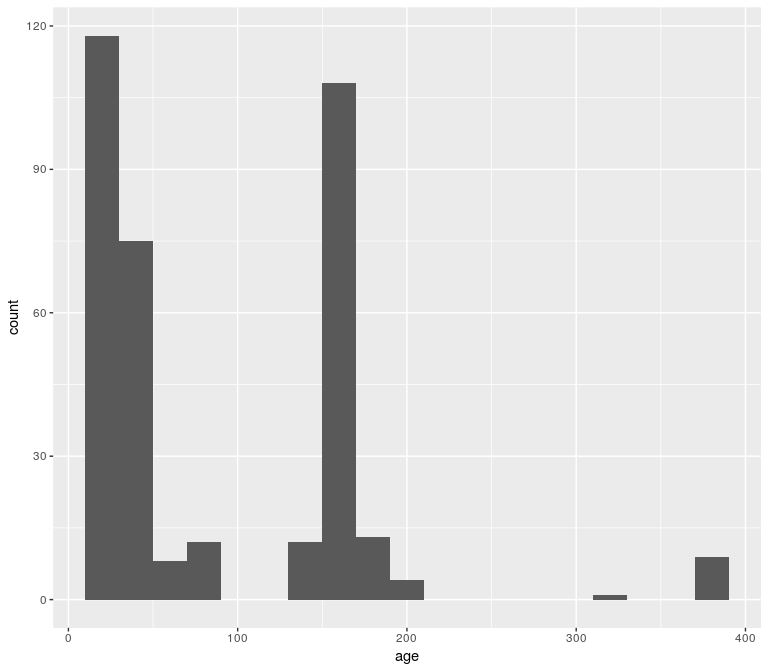Figure @ref(fig:preprocessing-setup) shows that the age covariate belongs to three separate clusters.

qplot(log10(rowSums(otu_table(ps))),binwidth=0.2) +
xlab("Logged counts-per-sample")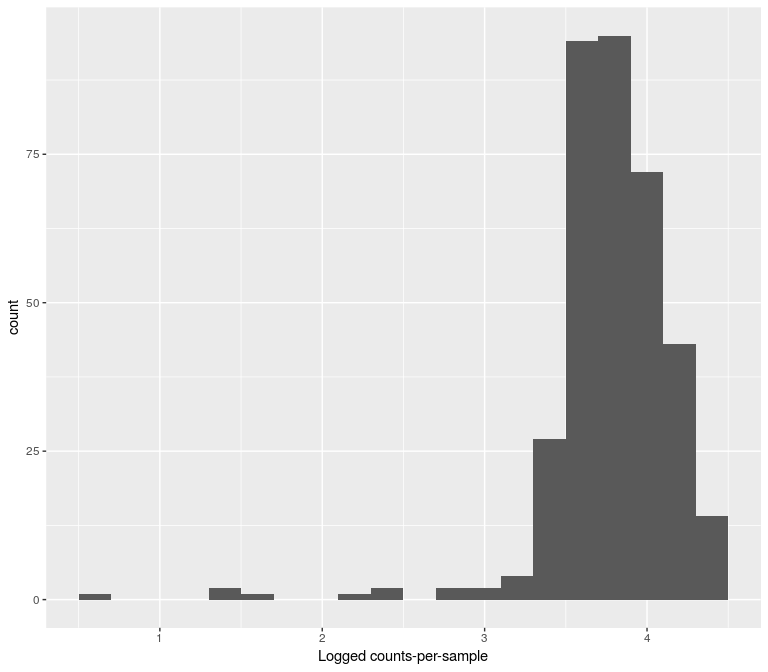These preliminary plots suggest certain preprocessing steps. The histogram in Figure @ref(fig:preprocessing-setup) motivates the creation of a new categorical variable, binning age into one of the three peaks.

The histogram in Figure @ref(fig:preprocessing2) suggests that a $$\log\left(1 + x\right)$$ transformation might be sufficient for normalizing the abundance data for the exploratory analyses.

In fact this transformation is not sufficient for testing purposes and when performing differential abundances we recommend the variance stabilizing transformations available in DESeq2 through the phyloseq_to_deseq2 function, see the phyloseq_to_deseq2 tutorial here.

As our first step, we look at principal coordinates analysis (PCoA) with either the Bray-Curtis dissimilarity on the weighted Unifrac distance.

sample_data(ps)$age_binned <- cut(sample_data(ps)$age,
breaks = c(0, 100, 200, 400))
levels(sample_data(ps)$age_binned) <- list(Young100="(0,100]", Mid100to200="(100,200]", Old200="(200,400]") sample_data(ps)$family_relationship=gsub(" ","",sample_data(ps)$family_relationship) pslog <- transform_sample_counts(ps, function(x) log(1 + x)) out.wuf.log <- ordinate(pslog, method = "MDS", distance = "wunifrac") evals <- out.wuf.log$values$Eigenvalues plot_ordination(pslog, out.wuf.log, color = "age_binned") + labs(col = "Binned Age") + coord_fixed(sqrt(evals / evals))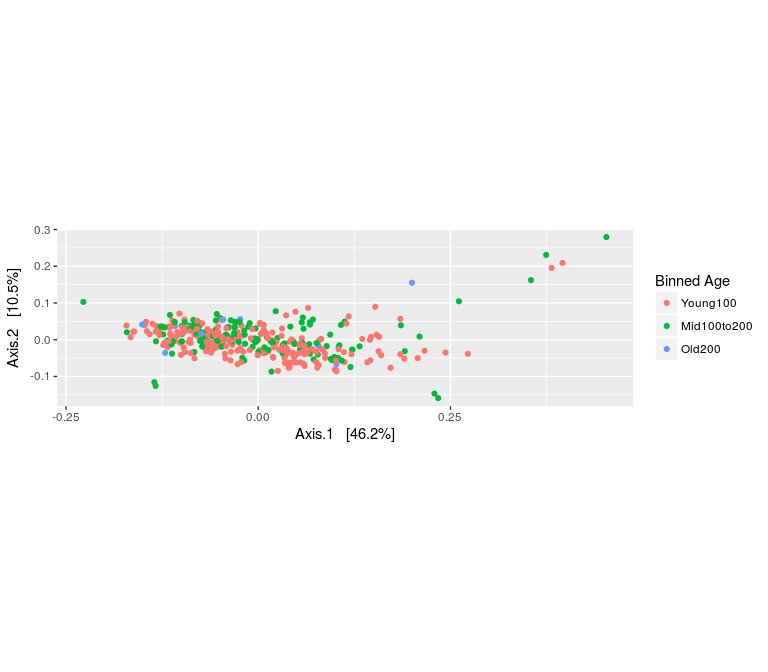Figure @ref(fig:outlier-detect) showing the ordination on the logged abundance data reveals a few outliers. These turn out to be the samples from females 5 and 6 on day 165 and the samples from males 3, 4, 5, and 6 on day 175. We will take them out, since we are mainly interested in the relationships between the non-outlier points. Before we continue, we should check the two female outliers – they have been taken over by the same OTU/ASV, which has a relative abundance of over 90% in each of them. This is the only time in the entire data set that this ASV has such a high relative abundance – the rest of the time it is below 20%. In particular, its diversity is by far the lowest of all the samples. rel_abund <- t(apply(otu_table(ps), 1, function(x) x / sum(x))) qplot(rel_abund[, 12], geom = "histogram",binwidth=0.05) + xlab("Relative abundance")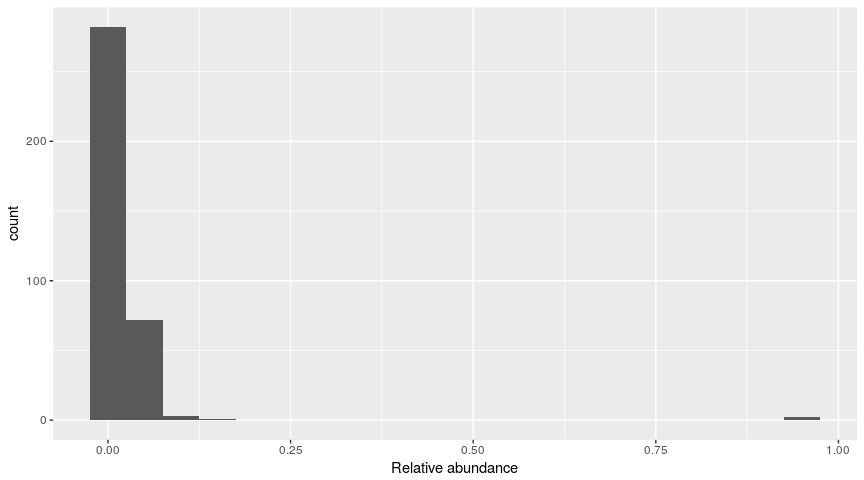# Different Ordination Projections As we have seen, an important first step in analyzing microbiome data is to do unsupervised, exploratory analysis. This is simple to do in phyloseq, which provides many distances and ordination methods. After documenting the outliers, we are going to compute ordinations with these outliers removed and more carefully study the output. outliers <- c("F5D165", "F6D165", "M3D175", "M4D175", "M5D175", "M6D175") ps <- prune_samples(!(sample_names(ps) %in% outliers), ps) We are also going to remove samples with fewer than 1000 reads: which(!rowSums(otu_table(ps)) > 1000) ## F5D145 M1D149 M1D9 M2D125 M2D19 M3D148 M3D149 M3D3 M3D5 M3D8 ## 69 185 200 204 218 243 244 252 256 260 ps <- prune_samples(rowSums(otu_table(ps)) > 1000, ps) pslog <- transform_sample_counts(ps, function(x) log(1 + x)) We’ll first perform a PCoA using Bray-Curtis dissimilarity. out.pcoa.log <- ordinate(pslog, method = "MDS", distance = "bray") evals <- out.pcoa.log$values[,1]
plot_ordination(pslog, out.pcoa.log, color = "age_binned",
shape = "family_relationship") +
labs(col = "Binned Age", shape = "Litter")+
coord_fixed(sqrt(evals / evals))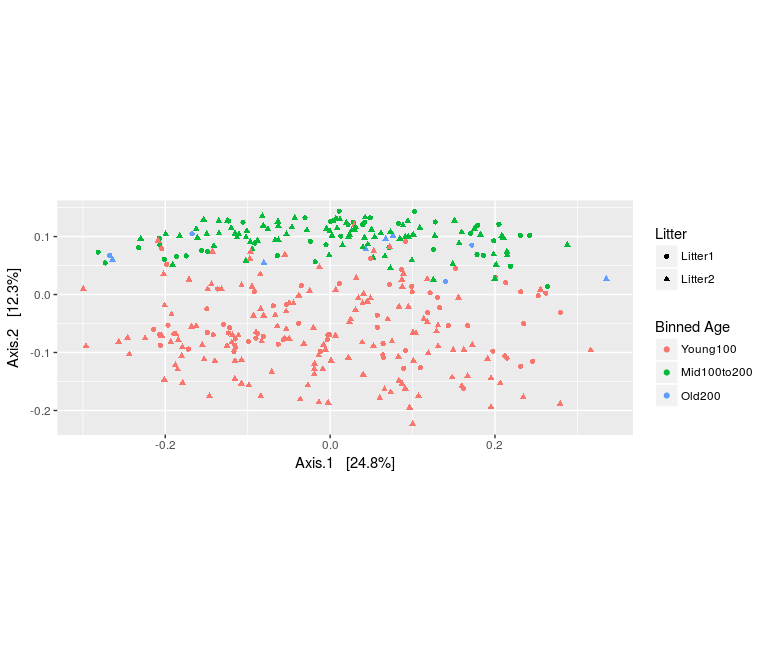We see that there is a fairly substantial age effect that is consistent between all the mice, male and female, and from different litters.

Next we look at double principal coordinates analysis (DPCoA) (Pavoine, Dufour, and Chessel 2004; Purdom 2010; Fukuyama et al. 2012), which is a phylogenetic ordination method and that provides a biplot representation of both samples and taxonomic categories. We see again that the second axis corresponds to young vs. old mice, and the biplot suggests an interpretation of the second axis: samples that have larger scores on the second axis have more taxa from Bacteroidetes and one subset of Firmicutes.

out.dpcoa.log <- ordinate(pslog, method = "DPCoA")
evals <- out.dpcoa.log$eig plot_ordination(pslog, out.dpcoa.log, color = "age_binned", label= "SampleID", shape = "family_relationship") + labs(col = "Binned Age", shape = "Litter")+ coord_fixed(sqrt(evals / evals))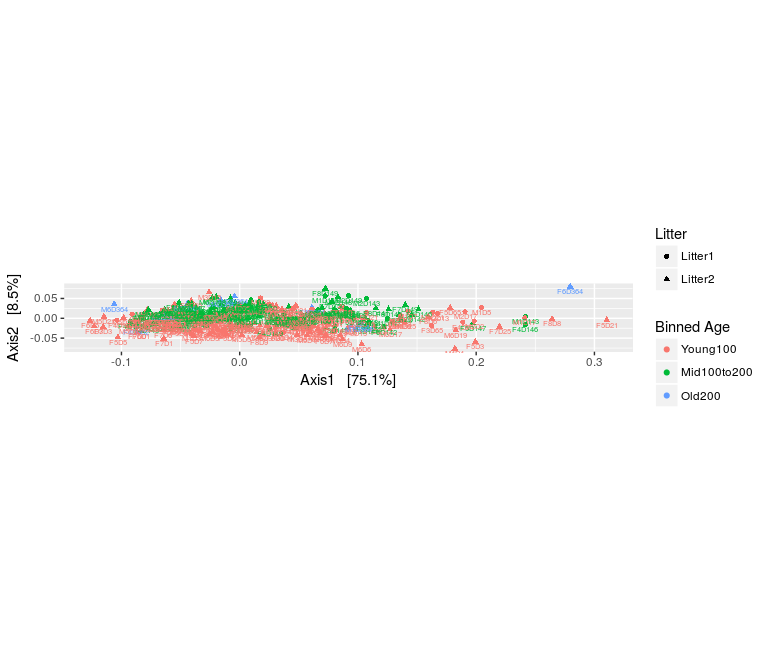In Figure @ref(fig:ordinations-dpcoa) we have the first axis explains 75 % of the variability, about 9 times that of the second axis; this translates into the elongated form of the ordination plot. plot_ordination(pslog, out.dpcoa.log, type = "species", color = "Phylum") + coord_fixed(sqrt(evals / evals))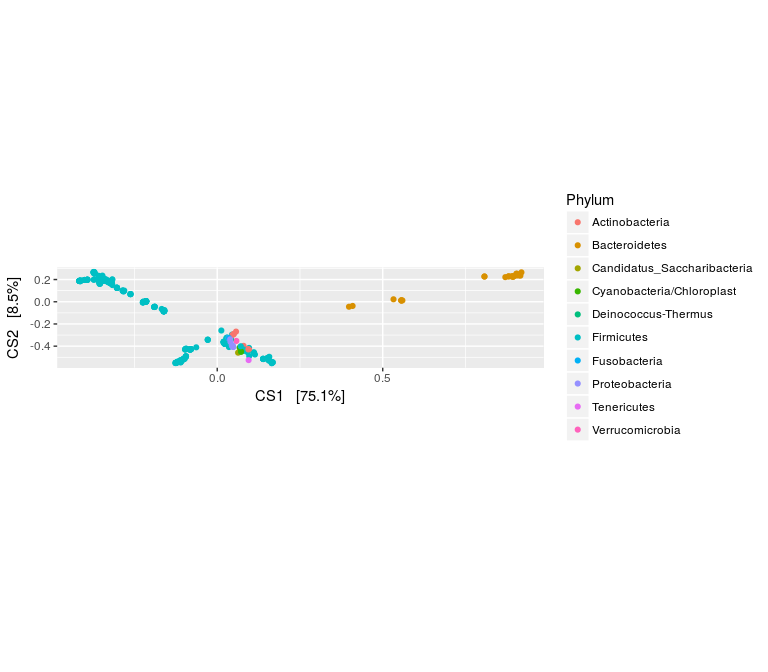Finally, we can look at the results of PCoA with weighted Unifrac. As before, we find that the second axis is associated with an age effect, which is fairly similar to DPCoA. This is not surprising, because both are phylogenetic ordination methods taking abundance into account. However, when we compare biplots, we see that the DPCoA gave a much cleaner interpretation of the second axis, compared to weighted Unifrac. out.wuf.log <- ordinate(pslog, method = "PCoA", distance ="wunifrac") evals <- out.wuf.log$values$Eigenvalues plot_ordination(pslog, out.wuf.log, color = "age_binned", shape = "family_relationship") + coord_fixed(sqrt(evals / evals)) + labs(col = "Binned Age", shape = "Litter")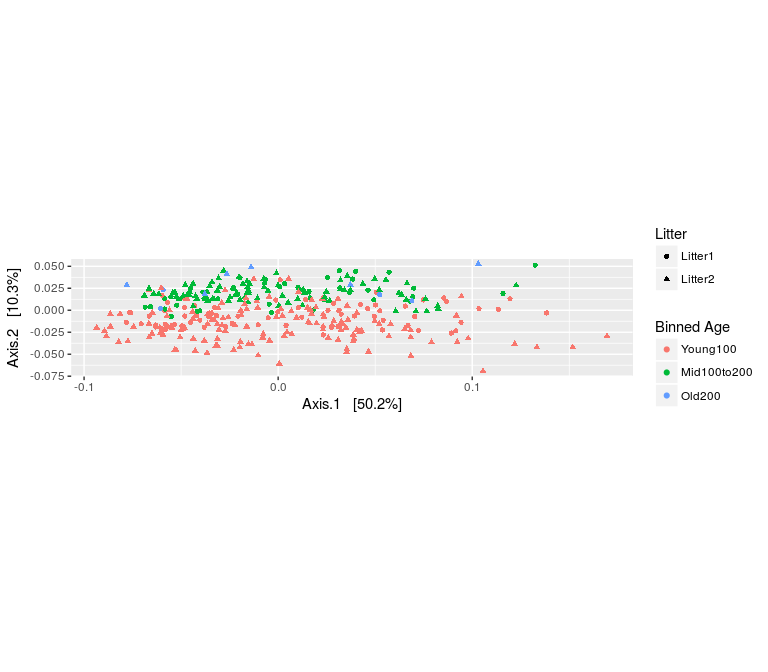## Why are the ordination plots so far from square? ### Aspect ratio of ordination plots In the ordination plots in Figure 8–Figure 14, you may have noticed as did the reviewers of the first version of the paper, that the maps are not presented as square representations as is often the case in standard PCoA and PCA plots in the literature. The reason for this is that as we are trying to represent the distances between samples as faithfully as possible; we have to take into account that the second eigenvalue is always smaller than the first, sometimes considerably so, thus we normalize the axis norm ratios to the relevant eigenvalue ratios. This ensures that the variability represented in the plots is done so faithfully. ### PCA on ranks Microbial abundance data is often heavy-tailed, and sometimes it can be hard to identify a transformation that brings the data to normality. In these cases, it can be safer to ignore the raw abundances altogether, and work instead with ranks. We demonstrate this idea using a rank-transformed version of the data to perform PCA. First, we create a new matrix, representing the abundances by their ranks, where the microbe with the smallest in a sample gets mapped to rank 1, second smallest rank 2, etc. abund <- otu_table(pslog) abund_ranks <- t(apply(abund, 1, rank)) Naively using these ranks could make differences between pairs of low and high abundance microbes comparable. In the case where many bacteria are absent or present at trace amounts, an artificially large difference in rank could occur(Holmes et al. 2011) for minimally abundant taxa. To avoid this, all those microbes with rank below some threshold are set to be tied at 1. The ranks for the other microbes are shifted down, so there is no large gap between ranks. abund_ranks <- abund_ranks - 329 abund_ranks[abund_ranks < 1] <- 1 library(dplyr) library(reshape2) abund_df <- melt(abund, value.name = "abund") %>% left_join(melt(abund_ranks, value.name = "rank")) colnames(abund_df) <- c("sample", "seq", "abund", "rank") abund_df <- melt(abund, value.name = "abund") %>% left_join(melt(abund_ranks, value.name = "rank")) colnames(abund_df) <- c("sample", "seq", "abund", "rank") sample_ix <- sample(1:nrow(abund_df), 8) ggplot(abund_df %>% filter(sample %in% abund_df$sample[sample_ix])) +
geom_point(aes(x = abund, y = rank, col = sample),
position = position_jitter(width = 0.2), size = 1.5) +
labs(x = "Abundance", y = "Thresholded rank") +
scale_color_brewer(palette = "Set2")